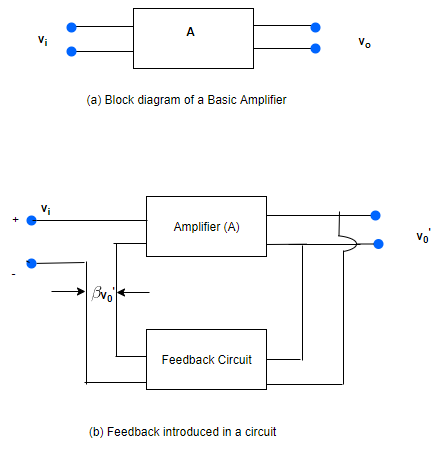# Feedback amplifiers

## Feedback amplifiers

• In a feedback amplifier the fraction of the amplifier output is fed back to the input circuit
• Feedback amplifiers consists of two parts
(i) An amplifier circuit
(ii) Feedback circuit
• Figure below shows how a feedback is introduced in an amplifier circuit• Figure(b) shows an amplifier without a feedback if vi and v0 are input and output voltage of the amplifier then voltage gain would be
A=v0/vi ---(13)

• Now consider figure (b) in which a feedback loop is added to the amplifier. If v0' is the output voltage with feedback and a fraction of its voltage β is applied to the input voltage then input voltage becomes vi+βv0' depending on whether the feedback voltage is in phase or out of phase with the input voltage

• If we consider the case of the positive feedback then input voltage becomes (vi+βv0') .When it is amplified by A times it becomes
A(vi+βv0')=v0'
or v0'(1-βA)=Avi
So the amplifier gain A' with feedback is given by

A'=v0'/vi =A/1-βA ---(14)
The above equation is positive feedback
• For negative feedback
A'=A/1+βA
and term βA is called feedback factor and A=v0/vi is called the internal gain of the amplifier
• From equation (14) and (15) we see that gain of amplifier increases when positive feedback is applied and decrease when negative feedback is applied
• For good performance of an amplifier ,we generally use negative feedback
• Positive feedback is used in making oscillator